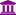# Is there a way to change names of various attributes at the same time?

Member Posts: 29Maven
I have created 20 attributes, and after that i realize that i want to have all the names of the attributes in uppercase, but if i change the name directly from Generate attribute operator i have to change all the functions that are correlated with attributes.

• Moderator, Member Posts: 999Unicorn
Hello @cdaponte

You can use "rename operator" or "rename by replacing operator" based on your attribute names.

• Member Posts: 29Maven
I have tried with the rename by replacing operator but i don´t have the results i want because each operator has a different name, so what i want to replace is different in each one.
• Administrator, Moderator, Employee, RapidMiner Certified Analyst, RapidMiner Certified Expert, University Professor Posts: 2,221RM Data Scientist
Hi,

i think the only way to do this is to take the XML and do Search and Replace in a text editor as of now.

BR,
Martin
- Head of Data Science Services at RapidMiner -
Dortmund, Germany
• Member, University Professor Posts: 1University Professor
I know that this is an older thread, and there is already an accepted answer, however, I had this issue and the above solution didn't work for me since my variable names were always changed.  I created a loop attributes with a rename and a macro variable inside.  Here is the xml using the titanic dataset.  Hope this helps someone.

<?xml version="1.0" encoding="UTF-8"?><process version="9.1.000">
<context>
<input/>
<output/>
<macros/>
</context>
<operator activated="true" class="process" compatibility="9.1.000" expanded="true" name="Process">
<parameter key="logverbosity" value="init"/>
<parameter key="random_seed" value="2001"/>
<parameter key="send_mail" value="never"/>
<parameter key="process_duration_for_mail" value="30"/>
<parameter key="encoding" value="SYSTEM"/>
<process expanded="true">
<operator activated="true" class="retrieve" compatibility="9.1.000" expanded="true" height="68" name="Retrieve Titanic" width="90" x="112" y="238">
<parameter key="repository_entry" value="//Samples/data/Titanic"/>
</operator>
<operator activated="true" class="rename_by_replacing" compatibility="9.1.000" expanded="true" height="82" name="Rename by Replacing" width="90" x="313" y="238">
<parameter key="attribute_filter_type" value="all"/>
<parameter key="attribute" value=""/>
<parameter key="attributes" value=""/>
<parameter key="use_except_expression" value="false"/>
<parameter key="value_type" value="attribute_value"/>
<parameter key="use_value_type_exception" value="false"/>
<parameter key="except_value_type" value="time"/>
<parameter key="block_type" value="attribute_block"/>
<parameter key="use_block_type_exception" value="false"/>
<parameter key="except_block_type" value="value_matrix_row_start"/>
<parameter key="invert_selection" value="false"/>
<parameter key="include_special_attributes" value="true"/>
<parameter key="replace_what" value="\W"/>
<parameter key="replace_by" value="_"/>
</operator>
<operator activated="true" class="concurrency:loop_attributes" compatibility="9.1.000" expanded="true" height="82" name="Rename with UpperCase" width="90" x="514" y="238">
<parameter key="attribute_filter_type" value="all"/>
<parameter key="attribute" value=""/>
<parameter key="attributes" value=""/>
<parameter key="use_except_expression" value="false"/>
<parameter key="value_type" value="attribute_value"/>
<parameter key="use_value_type_exception" value="false"/>
<parameter key="except_value_type" value="time"/>
<parameter key="block_type" value="attribute_block"/>
<parameter key="use_block_type_exception" value="false"/>
<parameter key="except_block_type" value="value_matrix_row_start"/>
<parameter key="invert_selection" value="false"/>
<parameter key="include_special_attributes" value="true"/>
<parameter key="attribute_name_macro" value="loop_attribute"/>
<parameter key="reuse_results" value="true"/>
<parameter key="enable_parallel_execution" value="true"/>
<process expanded="true">
<operator activated="true" class="generate_macro" compatibility="9.1.000" expanded="true" height="82" name="Generate Macro" width="90" x="112" y="34">
<list key="function_descriptions">
<parameter key="m_newname" value="upper(%{loop_attribute})"/>
</list>
</operator>
<operator activated="true" class="rename" compatibility="9.1.000" expanded="true" height="82" name="Rename (2)" width="90" x="246" y="34">
<parameter key="old_name" value="%{loop_attribute}"/>
<parameter key="new_name" value="%{m_newname}"/>
</operator>
<connect from_port="input 1" to_op="Generate Macro" to_port="through 1"/>
<connect from_op="Generate Macro" from_port="through 1" to_op="Rename (2)" to_port="example set input"/>
<connect from_op="Rename (2)" from_port="example set output" to_port="output 1"/>
<portSpacing port="source_input 1" spacing="0"/>
<portSpacing port="source_input 2" spacing="0"/>
<portSpacing port="sink_output 1" spacing="0"/>
<portSpacing port="sink_output 2" spacing="0"/>
</process>
</operator>
<connect from_op="Retrieve Titanic" from_port="output" to_op="Rename by Replacing" to_port="example set input"/>
<connect from_op="Rename by Replacing" from_port="example set output" to_op="Rename with UpperCase" to_port="input 1"/>
<connect from_op="Rename with UpperCase" from_port="output 1" to_port="result 1"/>
<portSpacing port="source_input 1" spacing="0"/>
<portSpacing port="sink_result 1" spacing="0"/>
<portSpacing port="sink_result 2" spacing="0"/>
</process>
</operator>
</process>